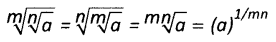# AP Board 8th Class Maths Notes Chapter 4 Exponents and Powers

Students can go through AP Board 8th Class Maths Notes Chapter 4 Exponents and Powers to understand and remember the concepts easily.

## AP State Board Syllabus 8th Class Maths Notes Chapter 4 Exponents and Powers

Laws of Exponents:

→ a × a × a …… m times = am

In am, a is called base; m is called exponent/ power.

→ am × an = am+n

→ $$\frac{\mathrm{a}^{\mathrm{m}}}{\mathrm{a}^{\mathrm{n}}}$$ = am-n (m > n)
⇒ $$\frac{1}{a^{n-m}}$$ (m < n)

→ (ab)m = am . bm

→ a0 = 1→ a-n = $$\frac{1}{a^{n}}$$

→ an = $$\frac{1}{a^{-n}}$$

→ $$\left(\frac{a}{b}\right)^{m}$$ = $$\frac{a^{m}}{b^{m}}$$

→ $$\left(a^{m}\right)^{n}$$ = amn→ $$\sqrt[n]{a}$$ = $$(\mathrm{a})^{1 / \mathrm{n}}$$